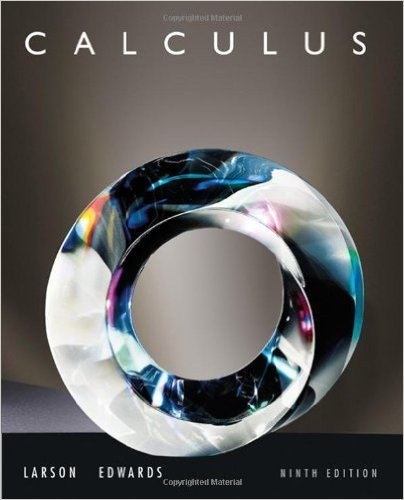×
×

# Solutions for Chapter 9.2: Series and Convergence## Full solutions for Calculus | 9th Edition

ISBN: 9780547167022Solutions for Chapter 9.2: Series and Convergence

Solutions for Chapter 9.2
4 5 0 306 Reviews
24
5
##### ISBN: 9780547167022

Chapter 9.2: Series and Convergence includes 143 full step-by-step solutions. Calculus was written by and is associated to the ISBN: 9780547167022. Since 143 problems in chapter 9.2: Series and Convergence have been answered, more than 61214 students have viewed full step-by-step solutions from this chapter. This textbook survival guide was created for the textbook: Calculus , edition: 9. This expansive textbook survival guide covers the following chapters and their solutions.

Key Calculus Terms and definitions covered in this textbook
• Additive inverse of a complex number

The opposite of a + bi, or -a - bi

• Blocking

A feature of some experimental designs that controls for potential differences between subject groups by applying treatments randomly within homogeneous blocks of subjects

• Constant of variation

See Power function.

• Directed line segment

See Arrow.

• Domain of validity of an identity

The set of values of the variable for which both sides of the identity are defined

• equation of a parabola

(x - h)2 = 4p(y - k) or (y - k)2 = 4p(x - h)

• First-degree equation in x , y, and z

An equation that can be written in the form.

• Half-plane

The graph of the linear inequality y ? ax + b, y > ax + b y ? ax + b, or y < ax + b.

• Initial value of a function

ƒ 0.

• Interval notation

Notation used to specify intervals, pp. 4, 5.

• Linear combination of vectors u and v

An expression au + bv , where a and b are real numbers

• Linear regression line

The line for which the sum of the squares of the residuals is the smallest possible

• Logarithmic regression

See Natural logarithmic regression

• Lower bound for real zeros

A number c is a lower bound for the set of real zeros of ƒ if ƒ(x) Z 0 whenever x < c

• Orthogonal vectors

Two vectors u and v with u x v = 0.

• Paraboloid of revolution

A surface generated by rotating a parabola about its line of symmetry.

• Pseudo-random numbers

Computer-generated numbers that can be used to approximate true randomness in scientific studies. Since they depend on iterative computer algorithms, they are not truly random

The graph in three dimensions of a seconddegree equation in three variables.

• Relation

A set of ordered pairs of real numbers.

• Standard form of a polynomial function

ƒ(x) = an x n + an-1x n-1 + Á + a1x + a0

×